# Hydrogen sulfide (H2S) Molecular geometry, electron geometry, SH2 lewis structure, Bond angle, dipole moment

Home  > Chemistry Article > H2S molecular geometry and it’s lewis structure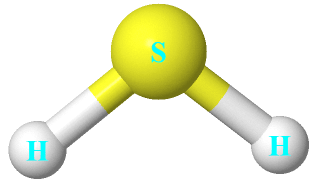Hydrogen sulfide is a colorless gas with a molar mass of 34.08 g·mol−1. It is poisonous and flammable with a smell like rotten gas. It is also dangerous for the environment.

In this article, we will discuss the following things- Hydrogen sulfide (H2S) molecular geometry,  lewis structure, electron geometry, its bond angle, polarity, hybridization, and dipole moment.

Hydrogen sulfide is produced by anaerobic digestion in which microorganisms break down the organic matter in the absence of oxygen. It also occurs in volcanic gases and in well water also. It can also be obtained by its separation from sour gas.

Hydrogen sulfide can be produced by when ferrous sulfide treats with a strong acid like HCl in standard lab preparation.

⇒ FeS + 2 HCl → FeCl2 + H2S

Most metals and non-metals sulfides after exposure to water they liberate Hydrogen sulfide.

⇒ 6 H2O + Al2S3 → 3 H2S + 2 Al(OH)3

H2S has many names like- Dihydrogen monosulfide, sewer gas, dihydrogen sulfide, sour gas, sulfane, sulfur hydride, etc.

Other properties of Hydrogen Sulfide

• H2S is slightly denser than air.
• The hydrogen sulfide chemical formula is H2S or SH2.
• It has a molar mass of 34.08 g·mol−1
• H2S has a boiling point of −60 °C and a melting point of −82 °C.
• It has a pungent smell, like rotten eggs.
• It has a vapor pressure of 1740 kPa.
• H2S magnetic susceptibility is −25.5·10−6 cm3/mol.
• Hydrogen sulfide act as a reducing agent.
• Its refractive index is 1.000644
 Name of Molecule Hydrogen sulfide Chemical formula H2S Molecular geometry of H2S Bent Electron geometry of H2S Tetrahedral Hybridization Sp³ Bond angle 92.1º Total Valence electron for H2S 8 The formal charge of H2S 0
Page Contents

## How to draw H2S/SH2 lewis structure and find it's hybridization?

H2S Lewis structure contains two hydrogens and one sulfur atom. Sulfur is the central atom and contains 2 lone pairs whereas both hydrogen is connected to the central atom with the help of a single bond.

Lewis’s structure of SH2 is really helpful to determine its electron geometry, molecular shape, number of shared pair, and lone pair electrons.

### Follow these steps to draw the lewis dot structure for H2S

Step 1: In the first step, determine the total valence electron present in H2S. As we know hydrogen only has one valence electron in its last shell and Sulfur belongs to the 16th group in the periodic table so it contains 6 electrons in its last shell.

⇒ Valence electron of hydrogen = 1×2 = 2

⇒ Valence electron of Sulfur = 6

∴ Total valence electron available for drawing the H2S lewis structure = 1×2 + 6 = 8 valence electronStep 2: Find the central atom for drawing the lewis dot structure for H2S. To determine the central atom in the H2S molecule you have two ways.

• Find the central atom by finding the least electronegative atom in the H2S molecule. Clearly, sulfur has less electronegativity compare to hydrogen because hydrogen has a higher tendency to attract electrons, also, hydrogen only needs two-electron to fulfill its valence shell.
• Place the atom at the center which has a greater valence. Clearly, Sulfur has a valence power of 6 and Hydrogen has only 1. So, Sulfur must be paced at the center in the lewis diagram.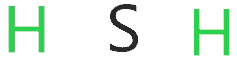Step 3: Now construct the structure of H2S by connecting the single bond to the central atom.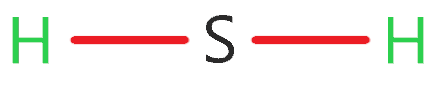Step 4: After placing the central atom and connecting with it a single bond, we have to fulfill the octet rule for every atom in H2S to maintaining the last requirement of the lewis diagram.

Hydrogen atom needs only two electrons to fulfill their octet and sulfur atom need eight electrons to fulfill the octet.

The hydrogen outer shell is already full because it shares two electrons already with the help of a single bond. So, no need to placing electrons around hydrogen.

Now for Sulfur, we need 8 electrons but only 4 electrons are shared with the help of two single bonds. So, we have to place 4 more electrons around sulfur to complete its octet rule and fulfilling the criteria for drawing the lewis dot structure for SH2.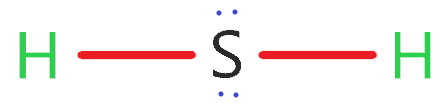### Lewis structure for SH2 or H2S

Step 5: Now in the final step, minimize the charges on atom of H2S by converting lone pairs to covalent bonds.

But there is no charge on the atom(formal charge on hydrogen and sulfur is zero), so, no need to make any covalent bond.

This lewis structure is also called Dihydrogen sulfide lewis structure or dihydrogen monosulfide lewis structure.

### Hybridization of H2S

To Calculate the hybridization of H2S Just follow 3 simple steps.

1. Find the number of atoms attached to the central atom. Clearly, only two atoms(hydrogen) are attached to the sulfur central atom.

2. Count lone pairs on that central atom. So, 4 lone pair electrons are present around sulfur, which means sulfur has two lone pairs.

3. Add the Number of attached atoms to the central atom + Lone pairs of that central atom

∴ 2 + 2 = 4

So, 4 means hybridization of H2S is Sp³.

## What are the electron and molecular geometry of H2S?

The molecular geometry of H2S is bent because the two lone pair electrons on sulfur central atom repel each other as well as adjacent bonded pair electrons, as a result, these electron pair(lone pair and bond pair) takes the position where the repulsion becomes minimum and they attains the stability(no further repulsion force exist in electrons pairs).

Hence, the final shape or molecular geometry of H2S appears like a bent structure or V-shaped.According to the VSEPR theory, to reduce the repulsion. the electron pairs move to maximum angle apart from each other and become stable.

Also, electron geometry for H2S is tetrahedral because 4 electrons which make 2 lone pairs around a sulfur atom are arranged in a tetrahedral geometry.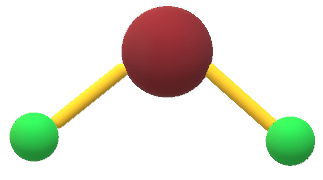### H2S molecular geometry or shape

To determine the electron and molecular geometry of H2S, its lewis structure and VSEPR theory play an important role.

Electron geometry considers all electrons as well lone pairs to determine the geometry of H2S whereas Molecular geometry only considers bonded atoms of the molecules to determine the shape of H2S.

## Another way to find electron/molecular geometry of H2S

AXN method is very useful to determine electron geometry or molecular shape for H2S. But you have to make a lewis structure of H2S for using this method.

AXN method

• A represents the central atom.
• X represents the bonded pair of electrons.
• N represents the lone pair of electrons.

As per H2S lewis structure –

• Sulfur is the central atom.
• 2 bonded pairs of electrons are attached to the central atom sulfur.
• 2 lone pairs on the sulfur central atom.

Therefore, the generic formula of hydrogen sulfide is AX2N2 for finding the molecular or electron geometry of H2S.

So, AX2N2 gives the molecular geometry of H2S is bent and the electron geometry is tetrahedral according to VSEPR Shape Chart.The bond angle of H2S is 92.1°.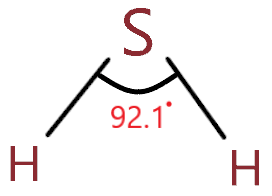## Is H2S polar or non-polar and its dipole moment

The polarity of H2S is dependent upon its molecular shape, electronegativity, and dipole moment.

H2S is non-polar because the electronegativity of bonds(H-S) is lesser than 0.5, as Hydrogen electronegativity is 2.20 and sulfur electronegativity is 2.58 and their difference is 0.38 which causes H2S to become nonpolar in nature.

According to the Pauling scale, if the difference of an atom’s electronegativity is less than 0.4, the bond is considered nonpolar and vice-versa.

H2S can also assume to be a polar molecule because it is non-linear and it has bent molecular geometry and central atom S is more electronegative than H, therefore, the negative pole is on the S atom. So, H2S has some dipole moment which makes it a polar molecule.

Technically, due to the very weak polarity nature of H2S, it is considered a non-polar molecule.

The dipole moment of H2S is 0.95D and in the mathematical term, it can be expressed as-

product of charges of two atoms and the distance between them

D = Q * R

⇒ D denotes dipole moment

⇒ Q denotes charge on atoms

⇒ R denotes the distance between atoms

Uses of Hydrogen Sulfide

• It is used to produce sulfur and sulfuric acid.
• It is used to produce pesticides, leather, and pharmaceuticals.
• It is also used in nuclear power plants for the production of heavy water.
• H2S is used in chemical analysis.
• It can also be used in agriculture as a disinfectant.

## FAQ

### Why is the bond angle in H2O greater than H2S?

This is because oxygen is more electronegative than the sulfur atom, hence, it will attract more electrons towards itself that cause more bond pair-bond pair repulsion between the bonds, and this finally causing the widening of bond angle in H2O.

∴ The bond angle of H2O = 104.5°

∴ The bond angle of H2S = 92.1°

### Why the molecular geometry of H2S is bent whereas its electron geometry is tetrahedral?

As we already discussed, “electron geometry is determined with the help of both lone electron pairs and bonds pair in a molecule whereas molecular geometry determined using only the bonds present in the molecule”.

According to the H2S lewis structure, the central atom has 2 lone pairs and 2 bonded pairs, hence, according to the VSEPR theory, H2S has 4 regions of density(2 lone pairs + 2bond pairs) around the central atom(sulfur).

∴ Four regions of electron density always form a tetrahedral geometry, hence, the electron geometry of H2S is tetrahedral.

While calculating the molecular shape, we will not consider the lone pair as “the molecular shape has to do with the shape of the actual molecule, not the electrons, so they are not accounted for in that sense”.

But we can’t neglect the effect of lone pair on a bond angle while calculating the molecular shape of the molecule.

As there are two lone pairs present on the sulfur central atom in the H2S molecule, hence, it will contract the bond pair, and this makes its shape appears like a bent structure.

Therefore, the molecular geometry or shape of H2S is bent while its electron geometry is tetrahedral.

## Summary

So, We discuss various things about Hydrogen sulfide in this article. Take a quick overview of this-

• The bond angle of H2S is 92.1°.
• H2S is nonpolar or very weak polar in nature due to minimal polarity exist in H-S bonds as per the Pauling scale.
• The dipole moment of H2S is 0.95D.
• Hybridization of H2S is sp³.
• H2S molecular geometry is bent.
• H2S electron geometry is tetrahedral.
• The total valence electron available for drawing the lewis structure of H2S is 8.
• The lone pair in on the central atom is 2 according to the lewis structure of SH2/H2S.
##### Subscribe to Blog via Email

Join 2 other subscribers

Share it...